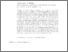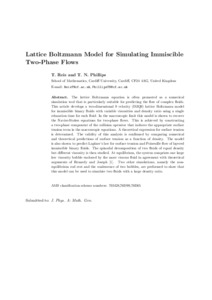Skip navigation

# Lattice Boltzmann model for simulating immiscible two-phase flows

and Phillips, T. N. (2007) Lattice Boltzmann model for simulating immiscible two-phase flows. Journal of Physics A: Mathematical and Theoretical, 40 (14). ISSN 1751-8113 (Print), 1751-8121 (Online) (doi:https://doi.org/10.1088/1751-8113/40/14/018)Preview
PDF (Author Accepted Manuscript)
23357 REIS_Lattice_Boltzmann_Model_for_Simulating_Iimmiscible_Two-Phase_Flows_2007.pdf - Accepted Version

Download (297kB) | Preview

## Abstract

The lattice Boltzmann equation is often promoted as a numerical simulation tool that is particularly suitable for predicting the flow of complex fluids. This paper develops a two-dimensional 9-velocity (D2Q9) lattice Boltzmann model for immiscible binary fluids with variable viscosities and density ratio using a single relaxation time for each fluid. In the macroscopic limit, this model is shown to recover the Navier–Stokes equations for two-phase flows. This is achieved by constructing a two-phase component of the collision operator that induces the appropriate surface tension term in the macroscopic equations. A theoretical expression for surface tension is determined. The validity of this analysis is confirmed by comparing numerical and theoretical predictions of surface tension as a function of density. The model is also shown to predict Laplace's law for surface tension and Poiseuille flow of layered immiscible binary fluids. The spinodal decomposition of two fluids of equal density but different viscosity is then studied. At equilibrium, the system comprises one large low viscosity bubble enclosed by the more viscous fluid in agreement with theoretical arguments of Renardy and Joseph (1993 Fundamentals of Two-Fluid Dynamics (New York: Springer)). Two other simulations, namely the non-equilibrium rod rest and the coalescence of two bubbles, are performed to show that this model can be used to simulate two fluids with a large density ratio.

Item Type: Article lattice Boltzmann, multiphase flow Q Science > QA Mathematics Faculty of Architecture, Computing & HumanitiesFaculty of Architecture, Computing & Humanities > Department of Mathematical Sciences 12 Apr 2019 15:42 None None None None http://gala.gre.ac.uk/id/eprint/23353

### Actions (login required)View Item

### Downloads

Downloads per month over past year

View more statistics